# How to generate random value from a given list in Excel?

This article is designed to allow users to understand the process and can generate random data values, from the provided list of data that is based on some predefined or provided data set or collection. Here, data can be of any type, for example, string, int, and any other. For this article will assume that the available data is of string type. The article contains two examples, first example performs the provided task by using the predefined formula. While the second example will perform the same task by using the kutool.

## Example 1 − To generate random value from a given list in Excel by using predefined formula.

### Step 1:

Consider below given Excel sheet with sample data to understand the method to generate random values from a given list in Excel sheet: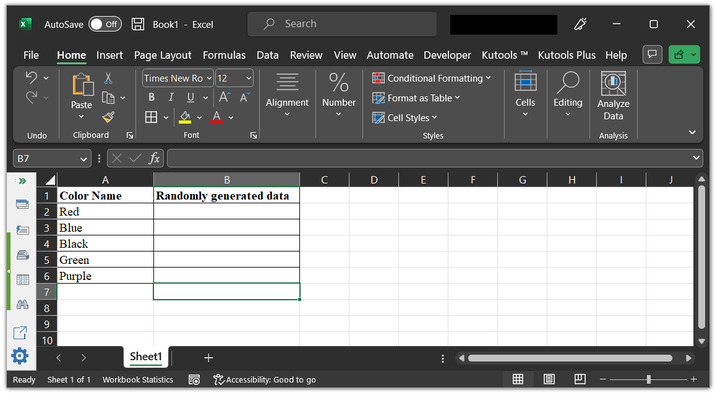### Step 2:

Go to the B2 cell and type formula “=INDEX($A$2:$A$6, RANDBETWEEN(2, 6))”.

### An explanation for the formula:

• INDEX() is a predefined Excel method. This method returns a reference value from the specified range.

• A2 to A6 cells are the range data cells.

• RANDBETWEEN() method is used to generate random numbers between the provided cell references, that is between row 2 and row 6. This function will pass a random index value to the INDEX() method.

• And, finally, INDEX() method, will read the index value and display the associated cell data on the worksheet.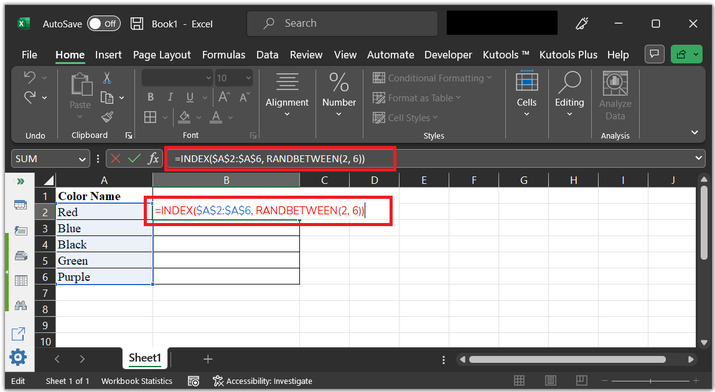### Step 3:

Press the “Enter” key. This will display a color name randomly on the B2 cell. consider below depicted image for proper reference: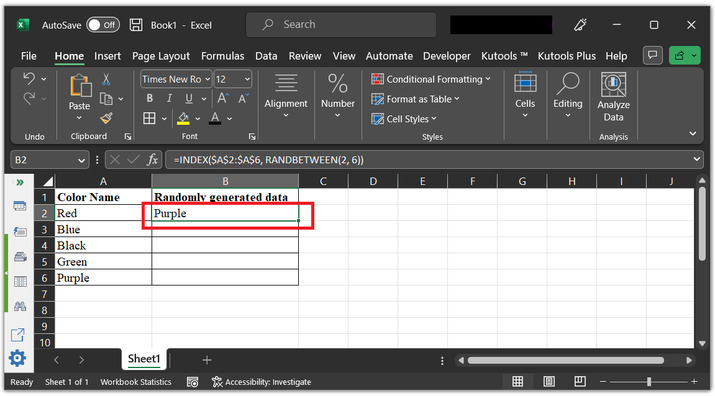Note − The same procedure will work for all the other data types such as int, string, and others as well.

## Example 2: To generate random value from a given list in Excel by using Kutool.

Step 1:

Consider the same Excel sheet again: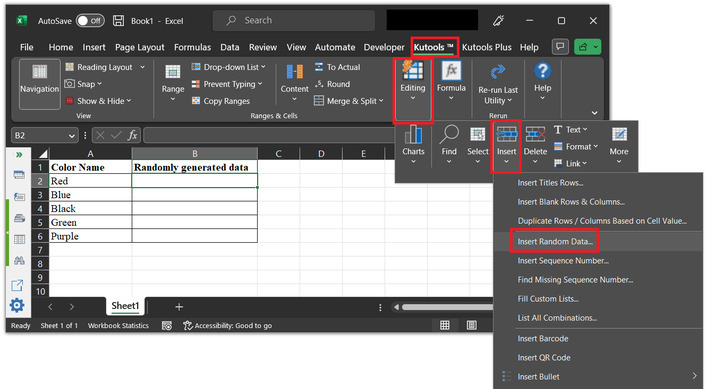### Step 2:

Go to the menu ribbon and click on the “Kutools” option. After that go to the “Editing” section. Choose the “Insert” option, and opt for the “Insert Random Data” option as shown in the below image.### Step 3:

This will open the “Insert Random Data” dialog box as shown below image: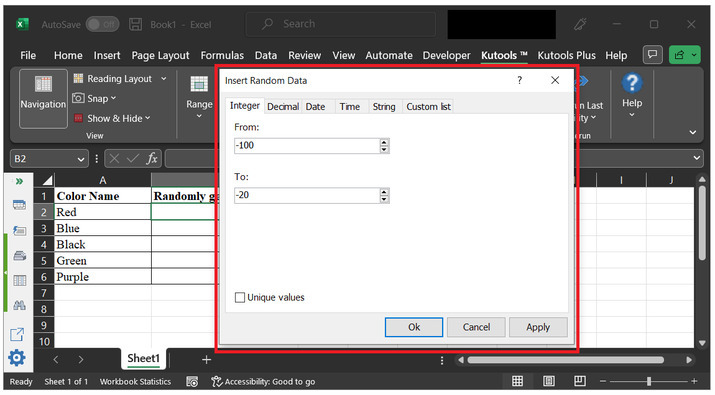### Step 4:

Select the last option tab “Custom list”. As the provided data is of string data type and user needs to add the data set into the list value, to generate a common random value from them. After that clock on the first “plus sign” option shown below, to add the data range.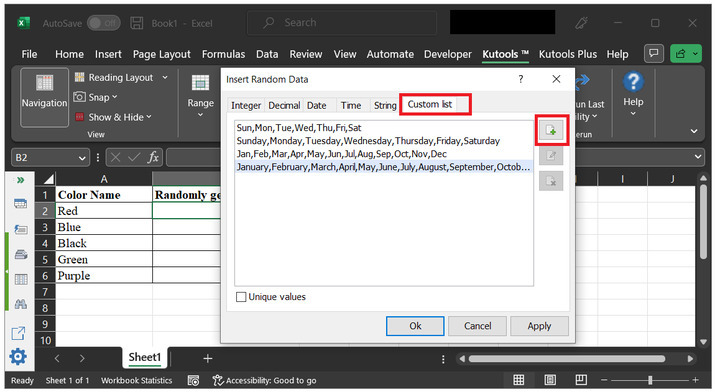### Step 5:

This will open the below given dialog box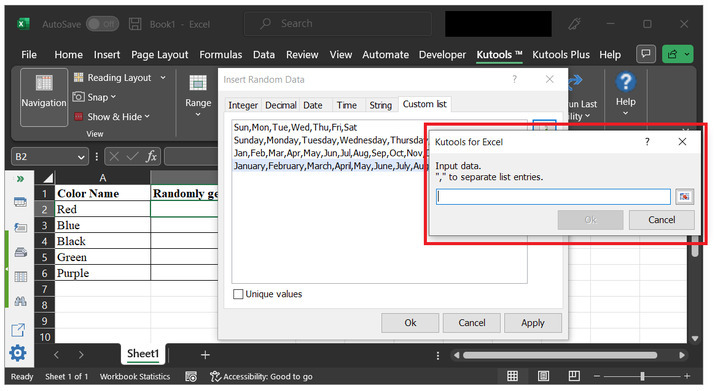### Step 6:

To add range, click on the side button, and use the mouse to select available data from the sheet.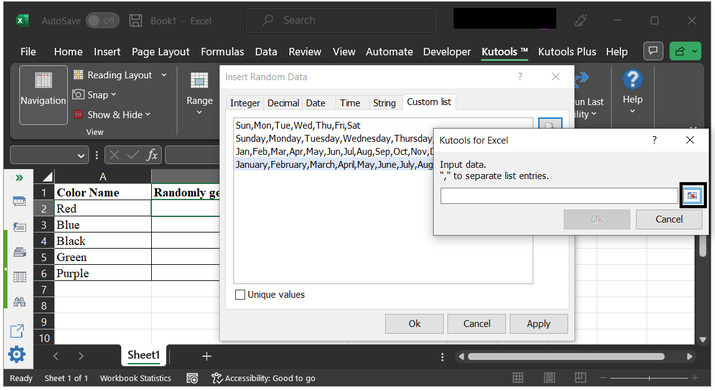### Step 7:

Use the mouse to select the data rows. The provided data range is A2 to A6. After that click on the “OK” button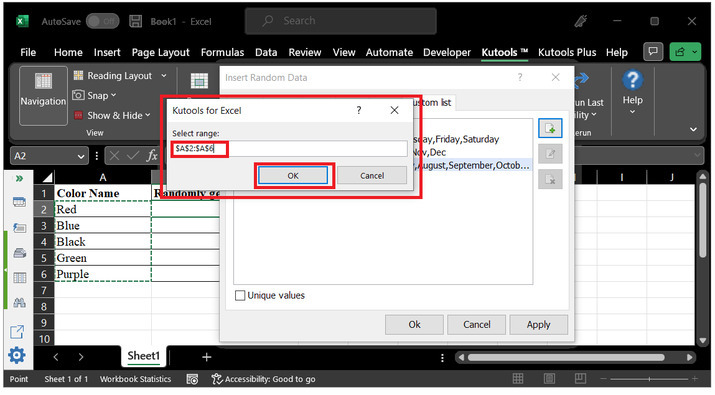### Step 8:

This will display the previous dialog box, again. But, this time the dialog box contains all the data values of the specified range. Simply click on “Ok” button.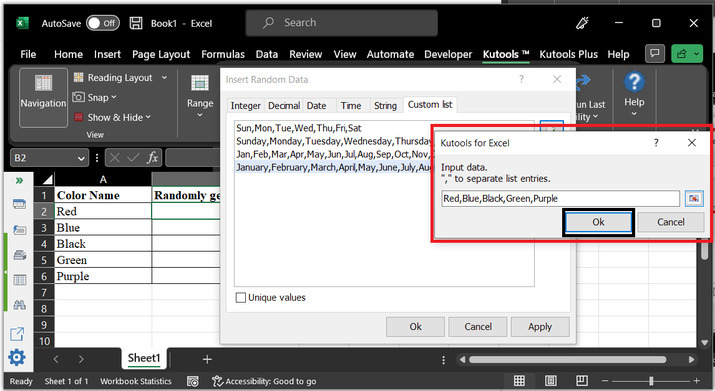### Step 9:

This will add the selected data to the custom list. Now, again click on “OK”.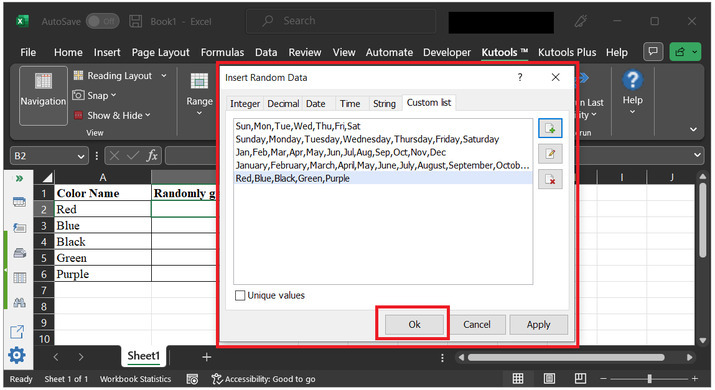### Step 10:

Random data values will be displayed on the console.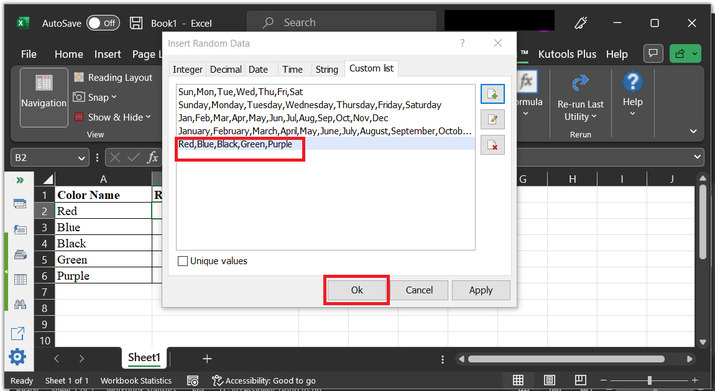### Step 11:

This will display a random data value on the active cell. consider below given output to understand the results properly: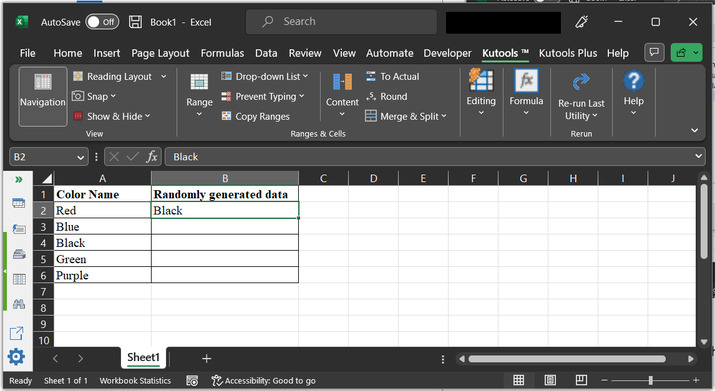## Conclusion:

After the successful completion of the above article, the user can easily understand the process of generating random data from the provided data set. In Excel, the same task can be done in multiple ways. But, in this article, the main focus is to guide the user about the most basic ways, by using which generating random data from the data set to become too easy and effective.

Updated on: 17-Apr-2023

385 Views